# RNA Identification Technique and RST Control of a Hybrid Indirect Matrix Converter with a Flying Capacitor Three Level Inverter in Power Active Filtering Application

RNA Identification Technique and RST Control of a Hybrid Indirect Matrix Converter with a Flying Capacitor Three Level Inverter in Power Active Filtering Application
Faculty of Technology, LACoSERE Laboratory, Amar Telidji University, Laghouat 03000, Algeria

Corresponding Author Email:
t.ameur@lagh-univ.dz
Page:
743-749
|
DOI:
https://doi.org/10.18280/jesa.540509
26 October 2020
|
Accepted:
10 August 2021
|
Published:
31 October 2021
| Citation

OPEN ACCESS

Abstract:

The present paper deals with RST controller using implementation of neural architectures to control power electronics systems dedicated to power quality improvement in a distribution grid. We present a technique for designing a robust RST controller, The computation of the controller is used to enhance the control a Hybrid Indirect Matrix Converter with a flying capacitor three level inverter (HIMC) coupled to the power grid (PG) by an RL filter, the synthesizing of this RST controller is formulated through open and close loop. Artificial neuronal network (ANN) identification technique is used to define/extract this disturbance. Simulation results by MATLAB, Simscap/sim power system code shows the effectiveness of the proposed method.

Keywords:

active power filtering, artificial neuronal network, flying capacitor inverter, indirect matrix converter, RST controller

1. Introduction

The quality of electrical energy concerns all actors in the energy sector, namely producers, distributors and consumers of electricity. It has become an important subject in recent years, because of electrical disturbances have a high cost for manufacturers because they cause production stoppages, losses of raw materials, a drop in the quality of production, premature aging of equipment. Power electronics, which are sensitive to disturbances or disturbance generators such as power switches .

Among the power electronic devices present on the electrical network, some converters are used in active filtering applications. Their action consists of inject currents into the network in order to improve the quality of voltages and currents available. Improving the performance of these devices under various conditions operation of energy sources and loads in the electrical network is a constant concern of certain players in the research world .

Harmonic current caused by non-linear loads reduce the PG quality. One of the several introduced solutions to compensate this harmonic is the active power filters (APF), The APF is composed of a transformer connects the grid to an AC-DC converter through a third order RLC filter , this converter feed a two capacitor filter to provide two separated voltage sources for the Flying Capacitor Inverter (FCI) and an RL filter.

This study is focused on the synthesize of an RST controller for the FCI and its output RL filter, we interest in the reference current tracking even when there is a perturbation on the FCI input DC voltage, for that reason, a transformer followed by a third order RLC filter as mentioned in Ref.  and a three phase full wave converter is used to provide a perturbed input DC voltage to the inverter. The FCI must generate an equal currant to the harmonics in an opposite phase, and it semi-conductors switches must support the voltage applied on it, switches dynamic represent the inverter time response, this dynamic has a reverse relation with the supported voltage .

To enhance the filtering quality an output passive filter recorded the inverter to PG is dimensioned to stop propagation of harmonics produced by the FCI to PG.

The FCI who belong to the serial multi-cellular inverter family had a topology that reduced the applied voltage on the switches, which let us pick a fast dynamic switch and allowed to used it in both middle and high PG voltage, moreover, these inverters harmonics are from rang fc×N (fc: carrier frequency; N: cell number) when we used carriers evenly phase shifted , and that reduced the constraints on the output filter. In this case a simple RL filter can satisfy the passive filter objective.

The commonly harmonics identification technique used is that named phase-locked loop (PLL) , as it exists several other methods such as wavelet , Kalman filtering (KF) , instantaneous power \cite{b5}, synchronous reference frame , fast Fourier transformation [9, 10] and ANN [11, 12].

An ANN of Adaline type which has an excellence performance in signal estimation  is introduced to extract the harmonic currant, this ANN type is proposed and developed by Widrow and Walach , and targets are the Fourier components amplitude of the harmonic currant.

This work is organized as follows: section Ӏ discusses in brief the active filtering process structure and parts; section IӀ for the RST controller synthesize method which done in the open and closed loop; section III results, discussion and comparison between both synthesize methods; section IV conclusion.

The Flying Capacitor Multilevel Inverter (FCMI) is considered as a regulated voltage source that is controlled in an open loop with the saw-tooth rotation PWM (STRPWM), it is considered as a first order system with less than one cycle of PWM duration delay, FCMI and its output RL filter represent a second order system which is controlled in a closed loop with an RST controller, the reference of this last loop is delivered by Adaline identification technique, full loop concept is schematized in Figure 1.

## 1.png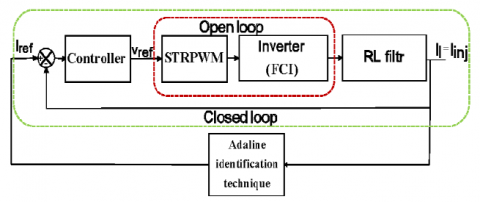Figure 1. Control structure

2. Active Power Filtering Structure

2.1 General structure

Figure 2 shows the current filtering process that composed of two parts, control part, starting from the measurement (both of current and or just the current), then an ANN of Adaline type identification technique extracts the harmonics components which named iref, a control law designed to guarantee the error elimination between iinj (injected current) and iref by generating the inverter switches duty cycle, after that a STRPWM is used to generates the inverter control signals and maintain the flying capacitors voltages. Power part contains the transformer with its filter, three phase rectifier, FCMI and its output RL filter.

## 2.png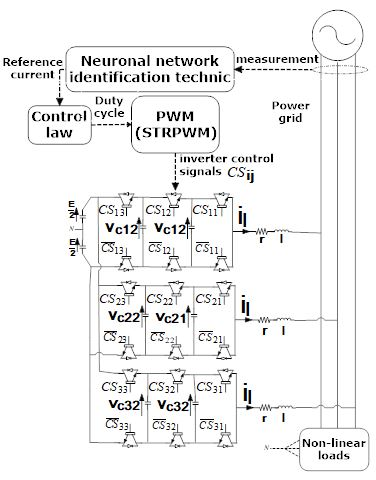Figure 2. Full system

ANN of Adaline type contains just an input layer and an output layer with a linear activation function as Figure 3 shows, the input vector X(t) is composed of functions that are used in the estimation of yref(t). Weights of the inputs are online adjusted by Widrow-Hoff algorithm modified by Nakajima and Masada  to minimize the error between output signal yesti(t) and yref(t). Fourier series equation of PG current is:

${{i}_{g}}(t)\,={{i}_{f}}(t)+{{i}_{h}}(t)$      (1)

When the fundamental component is:

${{i}_{f}}(t)\,={{i}_{11}}\cos (\omega t)+{{i}_{12}}\sin (\omega t)$            (2)

and the harmonics components is:

${{i}_{h}}(t)\,=\sum\limits_{k=2..M}{[{{i}_{k1}}\cos (k\omega t)+{{i}_{k2}}\sin (k\omega t)]}$            (3)

ω is the PG pulsation and M is the max range of harmonic to identified.

For our application, the input vector is:

$\begin{gathered} X(t) \\ =[\cos (\omega t), \sin (\omega t), \ldots, \cos (M \omega t), \sin (M \omega t)] \end{gathered}$            (4)

where, weights represent the amplitude of Fourier series components:

$W(t)=[{{i}_{11}},{{i}_{12}},...,{{i}_{M1}},{{i}_{M2}}]$            (5)

Widrow-Hoff modified algorithm is explained as:

W(t+k{{T}_{e}})=\left\{ \begin{align}& W(t)+\frac{\mu e(t)Y(t)}{X{{(t)}^{T}}Y(t)}\to X{{(t)}^{T}}Y(t)\ne 0 \\ & W(t)\to X{{(t)}^{T}}Y(t)=0 \\\end{align} \right.                 (6)

When

$Y(t)=\frac{1}{2}(sign(X(t))+X(t))$                (7)

and µ is the learning parameter, it defines the identification speed, but also its effects on the error e(t), a big value of µ gives a quickly identification with an observable average value of e(t), and a small value of µ slows down the identification and eliminate e(t), to profit this both advantages µ can be chosen variable during the transitional phase.

## 3.png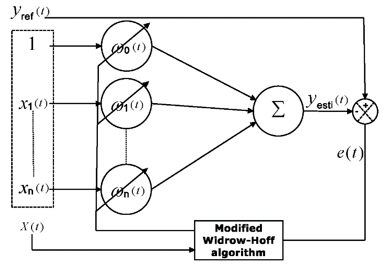Figure 3. ANN of Adaline type

2.3 Current control loop

As we mentioned previously, our full controlled system composed of the FCMI and an RL output filter, and because of the FCMI dynamic behavior, this inverter must be controlled and it control could be done in both closed or open loop in order to balance the flying voltages and generate the desired average voltage, control loop is presented in Figure 4, in both cases inverter transfer function is:

${{f}_{1}}(s)=\frac{1}{s+\tau }$            (8)

τ present the inverter delay. And the RL output filter TF is:

${{f}_{2}}(s)=\frac{1/l}{s+r/l}$            (9)

## 4.png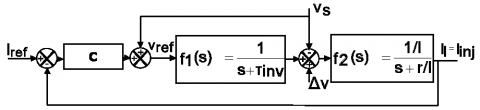Figure 4. Control loop

2.3.1 FCMI closed loop control

Several controller as PI [14, 15], predictive control [16, 17] and sliding mode  can satisfy the aims by using the state vector X for a single phase:

$\overset{\bullet }{\mathop{X}}\,(t)={{[\overset{\bullet }{\mathop{{{V}_{c1}}}}\,,\overset{\bullet }{\mathop{{{V}_{c2}}}}\,,\overset{\bullet }{\mathop{{{i}_{inj}}}}\,]}_{T}}$                  (10)

However, it needs complicated and precise calculations.

2.3.2 FCMI open loop control

One from the several solutions of the FCMI open loop control is PWM technique, it has been studied by Kang et al.  and gives for example phase shifted PWM (PSPWM), carrier redistributed PWM (CRPWM), modified CRPWM (MCRPWM) and saw tee rotation PWM (STRPWM). For the flying voltages (FV), this PWMs provides just the maintain not the convergence, which mean that the inverter must start working with a balanced voltage, to compensate this drawback, a simple balancing algorithm as it proposed by Defay  is applied in the beginning to balance this voltages. In a study done by Kang et al.  between PSPWM, MCRPWM and STRPWM, the last one provides the best tradeoff between THD and FV ripple, for this reason we used it in our study.

3. Control Method

An RST linear controller is implemented in order to eliminate the error between Iref(t) and Iinj(t). This polynomial controller provides more freedom degree then the classical PI controller . The transfer function (TF) of the loop shown in Figure 5.

## 5.png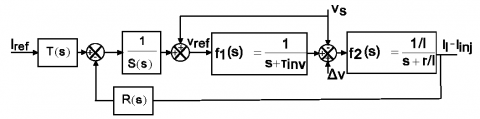Figure 5. Control loop with RST controller

\begin{align} & {{I}_{inj}}(s)=\frac{TB(S)}{(SA+RB)(s)}{{I}_{REF}}(s) \\ & +[\frac{S(S)}{(SA+RB)(s)}(\frac{1}{l}-(\frac{r}{l}+s))]{{V}_{s}} \\\end{align}          (11)

When A(s) and B(s) are the full system denominator and nominator respectively,

\begin{align} & A(s)=(s+\tau )(s+r/l) \\ & B(s)=1/(l\tau ) \\\end{align}       (12)

ΔV represent the inverter injected harmonic, and it frequency is fc*N which is filtered by the RL output filter. For that reason, we ignored it in the TF.

$D(s)=(SA+RB)(s)$              (13)

Eq. (13) is called Bezout equation. For the purpose of achieving regulation and robustness objectives we have:

The RST controller causality condition:

\begin{align} & Deg(S)\ge Deg(R) \\ & Deg(S)\ge Deg(T) \\\end{align}           (14)

As we have:

$Deg(A)\ge Deg(B)$            (15)

We got:

$Deg(D)=Deg(S)+Deg(A)$                 (16)

Perturbation (V(s)) rejection condition:

$Deg(S)=0$                 (17)

It is a simplified condition, for preciseness calculation of S(f)=0 we take f=50, f is the perturbation frequency.

Tracking condition:

$T(0)=R(0)$               (18)

The unknown parameters number (UPN) defined from Bezout equation:

$UPN=\deg (S)+\deg (R)+1$                 (19)

And for controlling all Bezout equation parameters:

$deg(R)\ge deg(A)$                    (20)

To get a unique solution:

$deg(R)=deg(A)=n$                (21)

For a (proper) controller:

$deg(S)=deg(R)$                 (22)

And it yields:

$deg(D)=2\times n$                  (23)

For a strictly proper controller we modify Eq. (22):

$deg(S)=deg(R)+1$              (24)

And it yields:

$deg(D)=(2\times n)+1$             (25)

In our application we chose a proper controller, and our strategy to define the unknown parameters is:

Elimination of system dynamic:

$deg(R)=deg(A)\Rightarrow deg(R)=2$                     (26)

And with no influence on the reference

$T(s)=R(s)$                 (27)

So the final loop with a rejected perturbation (V(s)) is illustrated in Figure 6:

## 6.png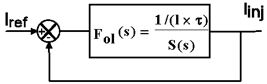Figure 6. The resulted equivalent control loop

Then we impose our desired dynamic:

$\deg (S)=2\Rightarrow S(s)={{s}_{2}}{{S}^{2}}+{{s}_{1}}S$                   (28)

S(s) parameters calculated either in an open loop (OL) as in a closed loop (CL).

First, CL controller synthesize: CL transfer function is:

${{F}_{cl}}(s)=\frac{1/(l\times \tau )}{{{s}_{2}}{{S}^{2}}+{{s}_{1}}S+1/(l\times \tau )}$                (29)

The desired denominator Ddesi(s) is:

${{D}_{desi}}(s)={{S}^{2}}+2\xi {{\omega }_{0}}S+\omega _{0}^{2}$                (30)

where, ξ= 0:7 which represent the best tradeoff between the time response tr and the pulsation ω0;

ω0 is defined to satisfied the condition:

$-{{5}_{DB}}\ge {{G}_{DB}}\ge -{{10}_{DB}}$         (31)

[-10DB, -5DB] is the interval that gives the acceptable ratio between performance and frequency filtering (robustness),

${{G}_{DB}}={{\left| {{F}_{cl}}(j{{\omega }_{f}}) \right|}_{DB}}$            (32)

ωf  is the pulsation that we’re up to filtrate and it represent the harmonics produced by the inverter from range fc×N. It is complicated to calculate the last equation, so we used the asymptote P(s) of Fcl in high frequency,

$\left| P(j\omega ) \right|={{(\frac{{{\omega }_{0}}}{\omega })}^{2}}$           (33)

then we replaced ­|Fcl(ωf)| by |P(ωf)| in Eq. (32), and Eq. (31) we got:

${{s}_{2}}={{(\frac{1}{\omega _{0}^{2}l\tau })}^{2}}$           (34)

${{s}_{1}}=2\xi {{\omega }_{0}}{{s}_{2}}$             (35)

Second, OL controller synthesize: OL transfer function is:

${{F}_{ol}}(s)=\frac{1/(l\times \tau )}{{{s}_{2}}{{S}^{2}}+{{s}_{1}}S}$           (36)

We know that both open and closed loop had the same response in high frequency, for this reason we used the condition Eq. (31) same as in CL, but instead of Eq. (32) we have:

${{G}_{DB}}={{\left| {{F}_{ol}}(j{{\omega }_{f}}) \right|}_{DB}}$             (37)

Eq. (37) developed to:

${{s}_{2}}^{2}\omega _{f}^{4}+{{s}_{1}}^{2}\omega _{f}^{2}-{{(\frac{B}{{{G}_{DB}}})}^{2}}=0$            (38)

To reach tracking in finite time we have:

$\Delta {{\varphi }_{desired}}=180+{{\left| {{F}_{ol}}(j{{\omega }_{c}}) \right|}_{DB}}$            (39)

This gives:

$\frac{{{\omega }_{c}}{{S}_{2}}}{{{S}_{1}}}=\tan (90-\Delta {{\varphi }_{desired}})$             (40)

ωc is the pulsation of |Fcl(ωf)|DB intersection with 0DB line,

$\left| {{F}_{ol}}(j{{\omega }_{c}}) \right|=1$     (41)

And it developed to:

${{s}_{2}}^{2}\omega _{c}^{4}+{{s}_{1}}^{2}\omega _{c}^{2}-{{B}^{2}}=0$            (42)

The desired phase margin condition for an accepted time response:

$60{}^\circ \ge \Delta {{\varphi }_{desired}}\ge 45{}^\circ$           (43)

Eq. (42) admit one acceptable solution value of ωc,

$\omega _{c}^{2}=\frac{-{{s}_{1}}^{2}+\sqrt{{{s}_{1}}^{4}+4{{({{s}_{2}}B)}^{2}}}}{2{{s}_{2}}^{2}}$          (44)

the other solution is negative, which is not an acceptable value. ωcs2 is replaced by its value from Eq. (40) in Eq. (44),

\begin{align} & {{S}_{2}}=\frac{{{S}_{1}}^{2}\eta }{B} \\ & \eta =\tan (90-\Delta {{\varphi }_{desired}})\sqrt{1+{{(\tan (90-\Delta {{\varphi }_{desired}}))}^{2}}} \\\end{align}       (45)

Then we replace Eq. (45) in Eq. (38), it gives one acceptable solution for S12:

${{S}_{1}}^{2}=\frac{\frac{{{B}^{2}}}{{{\eta }^{2}}}+\sqrt{\frac{{{B}^{4}}}{{{\eta }^{4}}}+\frac{4{{B}^{4}}}{{{(\eta \times {{G}_{DB}})}^{2}}}}}{2\omega _{f}^{2}}$      (46)

It is clear that we start our calculations with s1 from Eq. (46), and then we passed to calculate s2 from Eq. (45).

4. Results of Simulation

For the power grid simulation, we used a three-phase voltage generator, rectifier as a nonlinear load, resistor load as a linear load and our active filter with it RL output filter. All simulation values placed in Table 1.

We chose one phase to present and explain our results.

Table 1. Simulation values

 Parameters Value Adaline learning parameter µ 1 Carrier frequency fc 12 khz Simulation period 1-7s Transformer Output filter r,l 10Ω, 0.5mh Flying capacitors 470µf DC capacitor 470µf Cell number N=3 Δφdesired 50

Figure 7 shows the Adaline ANN identification technique results, upper plot presents the grid current with its estimation, and the lower plot present the estimated current fundamental and harmonic components.

## 7.png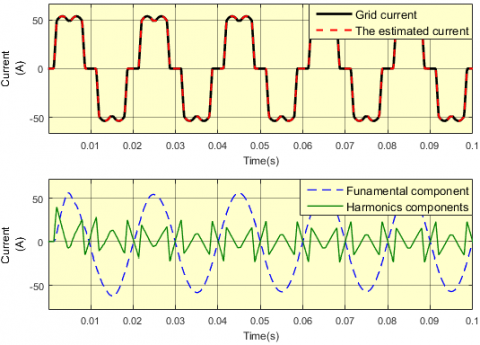Figure 7. Harmonics identification result

In term of response time, our interests focused on the harmonics identification time which is reached in the first half of fundamental period and that's made it very acceptable.

For the RST controller which synthesized in CL (RSTCL), Figure 8 presents Bode plot of closed loop, open loop and the asymptote transfer functions, and Figure 9 shows reference (estimated harmonic) tracking results.

The desired Reference tracking achieved even that we used an asymptote to avoid complicated calculations.

## 8.pngFigure 8. OL, CL and the asymptote Bode diagram

## 9.png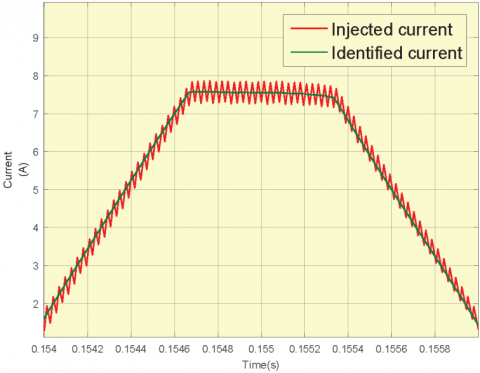Figure 9. Reference tracking when RST controller synthesized in CL

## 10.png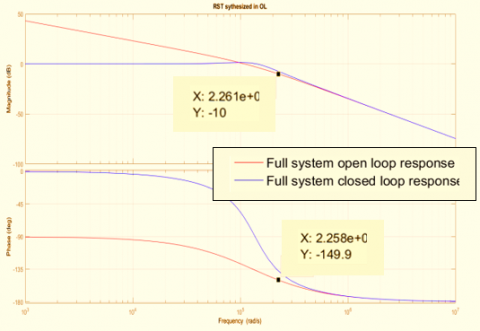Figure 10. OL and CL diagram

For the RST controller which synthesized in OL (RSTOL), Figure 10 presents Bode plot of closed loop and open loop transfer functions, and Figure 11 shows reference (estimated harmonic) tracking results.

Referring to the CL draws in both RST synthesize methods, the harmonic pulsation range that can be generated is from 2π(2×50) rad/s to 2pi(32×50) rad/s and that's a satisfy wide range, besides that, the inverter produced harmonic pulsation (2πfcN = 2.26×105) is clearly filtered. These two points present a good CL response.

A remarkable fast tracking with no delay is observed in Figure 9 and Figure 11, as it exists a small fast rippling that is interpreted buck to THD values. In term of simplicity, this method used the OL transfer function directly.

## 11.png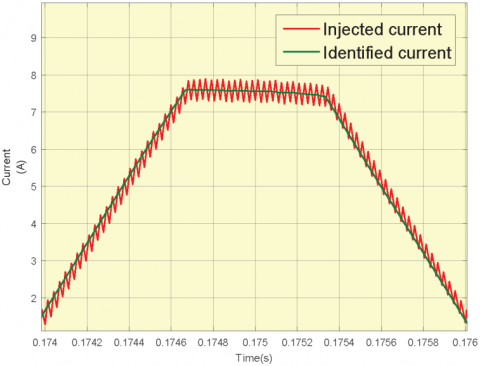Figure 11. Reference tracking when RST controller synthesized in OL

## 12.png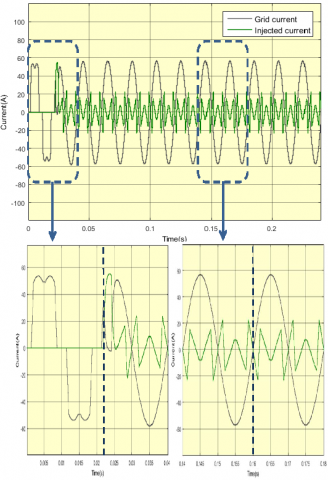Figure 12. Full filtering process

Grid current filtration operation results presented in Figure 12, filtration starts at t=0.02s and we used the RSTCL controller till t=0.16s then we switched to RSTOL as it is illustrated in the figure. While Figure 13.a shows THD (time harmonic distortion) value reduced from around 28% before filtration to 0.4% after filtration and that is observed from the sinusoidal form of the grid current obtained, and THD in Figure 13.b decrease again after switching the controller from the RSTCL to RSTOL at t=0.16s which explains the precise synthesis of RSTOL while in RSTCL synthesis we used approximation.

## 13a.png(a) THD during all operation

## 13b.png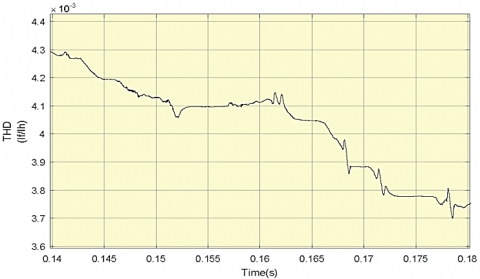(b) THD on the controller change interval (t=0.16s)

Figure 13. THD variation during filtering

5. Conclusion

In this paper a precise RST controller synthesize methods is presented, first method based on closed loop transfer function in addition of an asymptote, while the second method based on the open loop transfer function, both methods designed to satisfied fast harmonic tracking, robustness and rejects the FCMI switching harmonics. Also, the integration of the Adaline ANN shows an accurate and fast response to provide reference current to the controller. The maintain of the flaying capacitors voltages attains by using the STRPWM and the natural stability of the active power filter. Results obtained by simulation in MATLAB/Simscap/Sim Power System shows efficiency of these methods to ensure a reference finite time tracking in the filtration application.

Nomenclature
 fc carrier frequency, HZ R resistance, Ω L Inductance, H Greek symbols Δφ Phase margin, D° µ correction factor (learning factor) Subscripts N Cell number
References

 Mikkili, S., Panda, A.K. (2015). Power quality issues and solutions–review. International Journal of Emerging Electric Power Systems, 16(4): 357-384. https://doi.org/10.1515/ijeeps-2014-0052

 Maza-Ortega, J.M., Acha, E., García, S., Gómez-Expósito, A. (2017). Overview of power electronics technology and applications in power generation transmission and distribution. Journal of Modern Power Systems and Clean Energy, 5(4): 499-514. https://doi.org/10.1007/s40565-017-0308-x

 Azri, M., Rahim, N.A. (2011). Design analysis of low-pass passive filter in single-phase grid-connected transformerless inverter. 2011 IEEE Conference on Clean Energy and Technology (CET), Kuala Lumpur, Malaysia, pp. 348-353. https://doi.org/10.1109/CET.2011.6041489

 Allali, M.A. (2002) Contribution to the Study of Active Compensators for Low Voltage Electricity Networks, PHD thesis, Louis Pasteur – Strasbourg I University.

 Carrere, P. (1996). Study and realization of multicellular IGBT series converters, Balancing of floating capacitors. PHD thesis, Toulouse National Polytechnic Institute.

 Forghani, M., Afsharnia, S. (2006). Online wavelet transform-based control strategy for UPQC control system. IEEE Transactions on Power Delivery, 22(1): 481-491. https://doi.org/10.1109/TPWRD.2006.883026

 Kamwa, I., Grondin, R., McNabb, D. (1996). On-line tracking of changing harmonics in stressed power systems: application to Hydro-Quebec network. IEEE Transactions on Power Delivery, 11(4): 2020-2027. https://doi.org/10.1109/61.544290

 Guffon, S. (2000). Modeling and variable structure controls of active power filters. PHD thesis, Grenoble National Polytechnic Institute.

 Nakajima, T., Masada, E. (1989). An active power filter with monitoring of harmonic spectrum. EPE-89, 3rd European conference on power electronics and applications, Aachen, Germany.

 Naoussi, D.S.R. (2011). Implantation of Neuromimetic Networks on FPGA Target: Application to the Integration of an Active Filtering System, University of Strasbourg.

 Qasim, M., Kanjiya, P., Khadkikar, V. (2013). Artificial-neural-network-based phase-locking scheme for active power filters. IEEE Transactions on Industrial Electronics, 61(8): 3857-3866. https://doi.org/10.1109/TIE.2013.2284132

 Djaffar, O. (2005). Techniques neuromimétiques pour la commande dans les systèmes électriques: Application au filtrage actif parallµele dans les réseaux électriques basse tension. PHD thesis, Haute-Alsace University - Mulhouse.

 Widrow, B., Walach, E. (1996). Adaptive Inverse Control. Information and System Sciences Series. Prentice Hall Press, Upper Saddle River.

 Lott, C. (1995). Active parallel filtering of current harmonics on industrial networks: Theoretical study and production of a model at GTO, PHD thesis, Lorraine National Polytechnic Institute, Nancy, France.

 Xu, J. (1994). Filtrage actif parallèle des harmoniques des réseaux de distribution d’électricité. PHD Thesis, Lorraine National Polytechnic Institute, Nancy.

 Defay, F., Llor, A.M., Fadel, M. (2007). A direct predictive control of shunt active power filters using multicell converter. 2007 European Conference on Power Electronics and Applications, Aalborg, Denmark pp. 1-9. https://doi.org/10.1109/EPE.2007.4417411

 Defaÿ, F., Llor, A.M., Fadel, M. (2008). A predictive control with flying capacitor balancing of a multicell active power filter. IEEE Transactions on Industrial Electronics, 55(9): 3212-3220. https://doi.org/10.1109/TIE.2008.927989

 Said, S.B., Saad, K.B., Benrejeb, M. (2017). HIL simulation approach for a multicellular converter controlled by sliding mode. International Journal of Hydrogen Energy, 42(17): 12790-12796. https://doi.org/10.1016/j.ijhydene.2017.01.198

 Defay, F. (2008). Direct predictive control of a multicellular converter three phase for an active filtering application. PHD thesis, Toulouse National Polytechnic Institute, France.

 Kang, D.W., Lee, B.K., Jeon, J.H., Kim, T.J., Hyun, D.S. (2005). A symmetric carrier technique of CRPWM for voltage balance method of flying-capacitor multilevel inverter. IEEE Transactions on Industrial Electronics, 52(3): 879-888. https://doi.org/10.1109/TIE.2005.847574